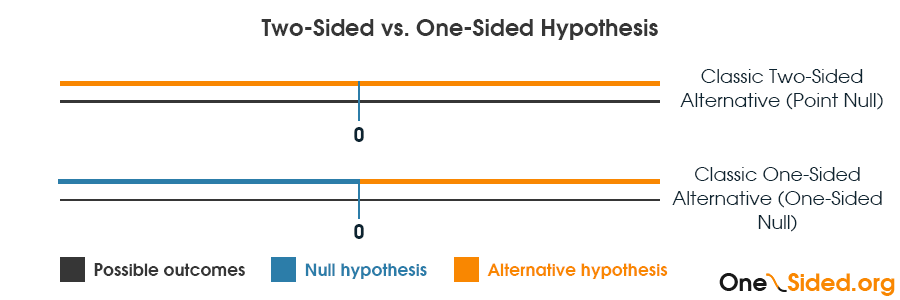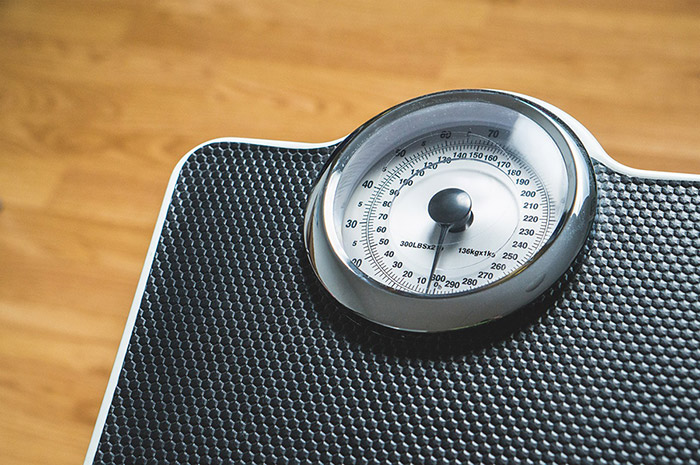# One-sided statistical tests are just as accurate as two-sided tests

Author: Georgi Z. Georgiev, Published: Aug 6, 2018

Since there are a lot of misconceptions and "bad press" about one-sided tests of significance and one-sided confidence intervals (for examples see "The paradox of one-sided vs. two-sided tests of significance" and "Examples of negative portrayal of one-sided significance tests") I want to set the record straight in this brief article. Namely, I will demonstrate that a one-sided test maintains its type I error guarantees just as well as a two-sided test and therefore refute claims about one-sided tests being biased, leading to more false positives or having more assumptions than two-sided tests.

## What is a one-sided test?

The p-value from a one-sided test of significance or the bound of an equivalent confidence interval is calculated under a null hypothesis that includes zero and one side of the distribution of possible outcomes of the measurement of interest. The alternative hypothesis covers just one side of the distribution as well. The above is the classical definition while in many practical scenarios a one-sided null hypothesis may span any proportion of the possible values of the outcome variable.Since the null of a one-sided test is broader it requires less data to reject it with the same level of uncertainty: in the classical scenario and a symmetrical error distribution we eliminate half of the possible outcomes from the pool of outcomes that can reject the null. This means that if for a two-sided hypothesis an error probability of 0.05 is maintained by setting the critical boundary cα = Z2 and -cα = -Z2, for a one-sided test the same error probability is maintained by the critical boundary cα = Z1 where Z1 < |Z2|.

Translated into the meaning of a p-value, observing a p-value of 0.01 from both a one-sided and a two-sided test means the same thing in terms of error probabilities: that were the null hypothesis true, we would observe such an extreme outcome, or a more extreme one, with probability 0.01. For a 99% one-sided ([llower; +∞) or (-∞; lupper]) or two-sided ([llower ; lupper]) interval it means that the true value will be within 99% of such intervals.

It should be noted that due the composite nature of the null hypothesis a one-sided test actually offers a conservative error guarantee, a maximum bound on the type I error. That is, if we have a null spanning from -∞ to 0, the reported p-value is calculated against the worst possible case: 0. If the null is in fact less than 0, then the true type I error can approach 0 for values significantly in the negative direction. This is exactly what we would if we want a claim to withstand even the most critical examination.

## There is nothing wrong in rejecting the null with outcomes in one direction only

I’ve seen the imprecise statement that by doing a one-sided test "we are looking in one direction only" Since a directional claim frames the alternative hypothesis as a one-sided one, in answering it we are limiting our rejection of the null hypothesis to outcomes in just one direction. For the purposes of rejecting a claim of no difference or negative (or positive) effect it is the only correct thing to do (See "Directional claims require directional hypotheses").

Making a claim of positive (or negative) effect is does not require one to predict, expect or hope for an outcome in that direction. Furthermore, predicting, expecting and hoping as experienced by the researcher have no effect on the true effect or the sampling space (possible experiment outcomes) and does not affect the data generating procedure in any way. No p-value adjustments or other precautions are necessary given a directional claim.

If one wants to examine the other direction they are free to do so. If one wants to entertain a more precise point null and calculate a two-sided p-value or confidence interval they are also free to do so, but it has no relevance to the research question that corresponds to a one-sided hypothesis.

## Explanation through a scale metaphorI find this weight measurement metaphor useful in illustrating the validity of a one-sided test versus a two-sided one. Say we have a scale and we know that 68.27% of the time it results in a measurement that is no more than ±1 kg off the true weight. Thus, assuming a normal distribution of error, the scale’s standard deviation is 1 kg. Suppose the allowed risk for any weight claim we make is 5%, corresponding to 1.644 kg critical value for a one-sided claim and 1.96 kg critical value for a two-sided claim.

We measure John’s weight and the scale shows 82 kg. Following this measurement, one can reject the claim "John weighs less than or equal to 80 kg" with a p-value of 0.0227 and the claim "John weighs exactly 80 kg" with a p-value of 0.0455.

Why is that?

Because under the second claim a scale indication of say 78 kg, which has a chance to happen due to random error even if John truly weighs exactly 80 kg, would also count against the null of "John weighs exactly 80 kg". The same 78 kg measurement would not count as evidence against the one-sided null of "John weighs less than or equal to 80 kg". In order for the p-value calculated relative to the "exactly 80 kg" to reflect the same error of probability as the "greater than 80 kg" claim it has to be adjusted to take into account those possible rejections that do not exist for the one-sided claim.

Note that we can just as easily state that we fail to reject the claim "John’s weigh less than 80 kg" with a p-value of 0.9773 (the one-sided test in the opposite direction) without any compromise of integrity or validity of the results.

The scale is obviously the statistical test of our choice and it remains uninterested in what claims any of us predicts, expects or wants to make after the weighing. The rest of the metaphor should be obvious.

## One- and two-sided hypothesis testing explained through statistical power

I always had an intuitive understanding of one-sided tests, but the details only downed on me once I examined the power functions of one-sided and two-sided tests of significance. It might not be as intuitive if you do not have a good grasp on the concept of power.

This is the graph I was looking at:Knowing that statistical power is the probability of rejecting the null with a given significance level if a given point alternative is true and that power function is plotting the power for a set of such alternatives we can understand a two-sided test with type I error 2α as two one-sided tests with type I error α, back to back. In fact, it appears that was how Fisher, Neyman & Pearson saw it as I discovered in writing "Fisher, Neyman & Pearson - advocates for one-sided tests and confidence intervals". The above assumes a symmetrical error distribution.

A statistical hypothesis that matches a directional research hypothesis is one in which we give up the ability to accept extreme results in one direction as rejecting the null based on the inquiry or claim of interest. In conducting a one-sided test matching such a hypothesis we give up power against all possible outcomes in the null direction and have zero power against many of them. The extent to which we give up power for true values under the null we are to decrease the reported probability of rejecting the null (p-value).

On the contrary, when doing a two-sided test, we add power by now accepting extreme results in the other direction as basis to reject the new, more precise point null. The power of the test under the null is doubled, so to maintain the same probability of a false rejection of the null we need to double the reported error (p-value) versus a test for a one-sided null bounded by the same value.

## Nothing works better in understanding statistics than a proper simulation

If you want to really learn statistics, do simulations. The easiest way to settle any disputes about the appropriateness and accuracy of a one-sided p-value is to see it through simulations.